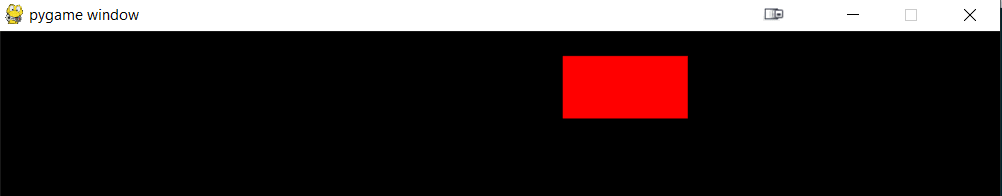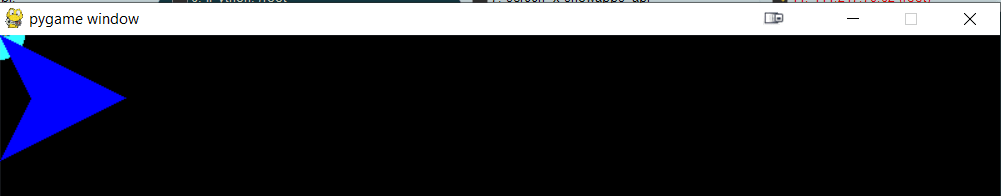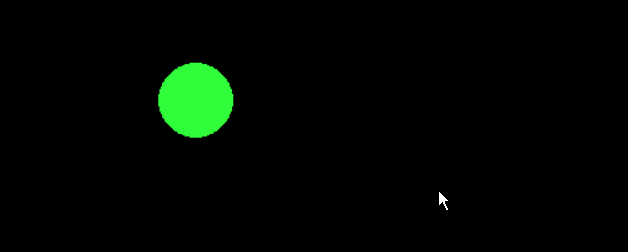# Learn Pygame With Examples

This post introduces pygame basic concepts. Before we procceed, make sure you have pygame library installed.

pip install pygame

First thing to do is to import the library and necessary packages.

In :
import pygame
import os
os.environ['SDL_AUDIODRIVER'] = 'dsp'

pygame 2.0.1 (SDL 2.0.14, Python 3.6.10)
Hello from the pygame community. https://www.pygame.org/contribute.html


## pygame.display()

Let us Initialize and Set the window size using set_mode() Once imported, we initialize pygame and we set up the window style and resolution. There are many ways to do it.

In :
WIDTH  = 800
HEIGHT = 600

WINDOW_SIZE = [WIDTH,HEIGHT]
pygame.init()
window = pygame.display.set_mode(WINDOW_SIZE)If we execute the snippet, a window with the size 800x600 will appear.

The function set_mode can have a second parameter "flag". Some of the most used flags are...

1. pygame.FULLSCREEN - To open the window in full screen mode
2. pygame.RESIZABLE -To allow the user to change the size of the window
3. pygame.NOFRAME - To have window with no border and no controls

1. pygame.SCALED - The window will adapt to the desktop size
2. pygame.SHOWN -The window will be visible (this is the default value)
3. pygame.HIDDEN - The window will open but will not be visible

Here is a simple example:

In [ ]:
import pygame
WINDOW_SIZE = [800,600]
pygame.init()
window = pygame.display.set_mode(WINDOW_SIZE,pygame.RESIZABLE)


We can use multiple flags at the same time like this...

In [ ]:
flags = pygame.SCALED | pygame.NOFRAME | pygame.HIDDEN
window = pygame.display.set_mode(WINDOW_SIZE,flags)


## pygame.QUIT()

Notice that after executing the previous code, you would see that Window Control bar status as "not responding". To fix this we need to add a loop and manage different events.

To capture the quit event, First we need to get the events and then compare each event in the event list with pygame.QUIT.

Here is a simple example...

In [ ]:
import pygame
pygame.init()
window = pygame.display.set_mode([800,600],pygame.RESIZABLE)
game_running = True

while game_running :
for event in pygame.event.get():
if event.type == pygame.QUIT:
print("closing the game")
game_running = False
break

pygame.quit()
exit()


Now with the above fix, we would'nt have the "not responding" problem and now we can interact with the window properly.

We can add any event to close the application such as "pressing the ESCAPE key" action.

To do that, we check for event pygame.K_ESCAPE as shown below...

In [ ]:
if event.type == pygame.KEYDOWN:
if event.key == pygame.K_ESCAPE:
game_running = False
break


If you want to explore all the available keys in Python, execute following help command. In the data section you will find all the keyboard keys starting with "K_"

In [ ]:
import pygame
help(pygame)


## Customize Display

We can set the background color & image as we want.

To set the color, we use RGB code.

In [ ]:
import pygame
import os
os.environ['SDL_AUDIODRIVER'] = 'dsp'

R = 200
G = 200
B = 200
grey = [R,G,B]

pygame.init()
window = pygame.display.set_mode([800,600])
window.fill(grey)

pygame.display.flip() # this is used to Save the changes to the window

run = True

while run :
for event in pygame.event.get():
if event.type == pygame.QUIT:
run = False
break
pygame.quit()
exit()


To set an Image as background, we need to make sure the resolution of the image is the same as our screen resolution.

First we load the image to a variable and then we add it to the window.

The function blit in the code below will display image starting at coordinates (0,0)

In [ ]:
import pygame

pygame.init()
window = pygame.display.set_mode([800,600])

#In this case we want image to be displayed in full screen so the top left corner of the image will be at the position coordinates (0,0)
window.blit(myBackgroundImage,(0,0))

pygame.display.flip() # this is used to Save the changes to the window

run = True

while run :
for event in pygame.event.get():
if event.type == pygame.QUIT:
run = False
break
pygame.quit()
exit()


## Changing Screen Tick Rate

If you want to add a tick rate to change the refresh rate of the screen, use following snippet.

In [ ]:
rate = 60
clock = pygame.time.Clock()  # this is added before the while loop
# instructions ...

while True :
clock.tick(rate) # this is set inside the while loop
# instructions ...


In [ ]:
import pygame

pygame.init()
window = pygame.display.set_mode([800,600])

# X and Y represent the position where the rectangle will be displayed

X = 20
Y = 20

# HEIGHT and WIDTH represent the size of the rectangle

WIDTH = 100
HEIGHT = 50

myRectangle = pygame.Rect(X,Y,WIDTH,HEIGHT)

# we can change the color of the rectangle before drawing

pygame.draw.rect(window,[200,200,200],myRectangle)

pygame.display.flip()

run = True

while run :
for event in pygame.event.get():
if event.type == pygame.QUIT:
run = False
break
pygame.quit()
exit()We can move the rectangle around by filling the window and drawing the rectangle again at another position.

In [ ]:
import pygame

rate = 60
clock = pygame.time.Clock()

pygame.init()
window = pygame.display.set_mode([800,600])

# X and Y represent the position where the rectangle will be displayed

X = 20
Y = 20

# HEIGHT and WIDTH represent the size of the rectangle

WIDTH = 100
HEIGHT = 50

myRectangle = pygame.Rect(X,Y,WIDTH,HEIGHT)

# we can change the color of the rectangle before drawing

pygame.draw.rect(window,[200,200,200],myRectangle)

pygame.display.flip()

run = True

while run :
for event in pygame.event.get():
if event.type == pygame.QUIT:
run = False
break

clock.tick(rate)
# fill the window with the color black ( if you have an image you will blit it again here)
window.fill([0,0,0])
X += 10 # here we change the position of the rectangle
myRectangle = pygame.Rect(X,Y,WIDTH,HEIGHT)
pygame.draw.rect(window,[255,0,0],myRectangle)
pygame.display.flip()

pygame.quit()
exit()### Adding a Circle to the Window

To add a circle to the window, follow the same steps as described above for the rectangle.

In [ ]:
position = (50,50)     # position of the center of the circle.
color = [50,255,255]   #color of the circle


In [ ]:
import pygame

pygame.init()
window = pygame.display.set_mode([800,600])

# X and Y represent the position where the rectangle will be displayed

position = (0,0)
color = [50,255,255]

pygame.display.flip()

run = True

while run :
for event in pygame.event.get():
if event.type == pygame.QUIT:
run = False
break
pygame.quit()
exit()


### Adding a Polygon to the Window

In [ ]:
color= [0,0,255]
positions = [(0,0),(50,100),(100,0),(100,50)]
pygame.draw.polygon(window, color, positions)

In [ ]:
import pygame

pygame.init()
window = pygame.display.set_mode([800,600])

# X and Y represent the position where the rectangle will be displayed

color= [0,0,255]
positions = [(0,0),(25,50),(0,100),(100,50)]
pygame.draw.polygon(window, color, positions)

pygame.display.flip()

run = True

while run :
for event in pygame.event.get():
if event.type == pygame.QUIT:
run = False
break
pygame.quit()
exit()If we want to move the circle, we have to change the coordiantes of the center.

If we want to change the position of polygon, we need to change all its coordinates.

Here is an example :

In [ ]:
def move_circle (delta_x,delta_y,position):
center = [position+delta_x,position+delta_y]
color = [50,255,60]
return center

def move_rectangle (delta_x,delta_y,pos):
moved = pos
moved+=delta_x
moved+=delta_y
myRectangle = pygame.Rect(moved,moved,50,25)
pygame.draw.rect(window,[250,65,65],myRectangle)
return moved

def move_polygon (delta_x,delta_y,positions):
moved = positions[::]
for e in moved :
e+=delta_x
e+=delta_y

pygame.draw.polygon(window, [0,0,255], moved)
return moved

import pygame
import time

pygame.init()
window = pygame.display.set_mode([800,600])

# X and Y represent the position where the rectangle will be displayed

color= [0,0,255]
positions = [[0,0],[25,50],[0,100],[100,50]]
pygame.draw.polygon(window, color, positions)

position = (20,150)
color = [50,255,60]

rect_coord =[0,200,50,25]
myRectangle = pygame.Rect(rect_coord)

# we can change the color of the rectangle before drawing

pygame.draw.rect(window,[250,65,65],myRectangle)

pygame.display.flip()

run = True

while run :
for event in pygame.event.get():
if event.type == pygame.QUIT:
run = False
break

window.fill([0,0,0])
position=move_circle(1,0,position)              # move the circle in the x direction (horizontally)
positions = move_polygon(1,0,positions)         # move the polygon in the x direction (horizontally)
rect_coord= move_rectangle(1,0,rect_coord)      # move the rectangle in the x direction (horizontally)
pygame.display.flip()

time.sleep(0.005)
pygame.quit()
exit()


Of course you can change the direction and the coordinates. I have used (1,0) as the first two parameters of the move_rectangle and move_polygon functions. we can set positive or negative values to navigate the shape over the window.

## Getting Mouse Position using pygame.mouse.get_pos()

To get the mouse position, it is very simple. we can use the function pygame.moust.get_pos()

In [ ]:
import pygame
import time

pygame.init()
window = pygame.display.set_mode([800,600])

run = True

while run :
for event in pygame.event.get():
if event.type == pygame.QUIT:
run = False
break
if event.type ==pygame.MOUSEBUTTONDOWN:
mouse_btn_pressed = True
print("mouse",event.button,"has been pressed")

position = pygame.mouse.get_pos()
print("mouse position",position)
time.sleep(0.5)
pygame.quit()
exit()## pygame Drag and Drop Game Example

Let us make a little drag and drop game.

If the user clicks a button, the circle will follow the mouse and if the mouse button is clicked again the circle will stop following.

In [ ]:
import pygame

pygame.init()
window = pygame.display.set_mode([800,600])

drag_drop = False
run = True

position = (400,300)
color = [50,255,60]
pygame.display.flip()

while run :
for event in pygame.event.get():
if event.type == pygame.QUIT:
run = False
break
if event.type ==pygame.MOUSEBUTTONDOWN:
mouse_btn_pressed = True
drag_drop = not drag_drop

if (drag_drop):
position = pygame.mouse.get_pos()
window.fill([0,0,0])
pygame.display.flip()
pygame.quit()
exit()## Displaying Text on the Screen using window.blit()

To display the text on the screen we need to follow these steps :

1 - set a font using : font = pygame.font.Font(pygame.font.get_default_font(), 36)

2 - set the text using : text = font.render('Hello World', True,255, 255, 255)

3 - display it on the screen using : window.blit(text,dest=(50,50))

In [ ]:
# here is a little example.
import pygame

pygame.init()
window = pygame.display.set_mode([800,600])

font = pygame.font.Font(pygame.font.get_default_font(), 36)
text = font.render('Hello World',True, (255, 255, 255))

run = True

window.blit(text,dest=(50,50))

pygame.display.flip()

while run :
for event in pygame.event.get():
if event.type == pygame.QUIT:
run = False
break

pygame.quit()
exit()


We can change the text displayed on the screen by filling the screen with the background color and blit'ing another text. And Don't forget to update the window using the function pygame.display.flip()